Courses

# Fuel Gas Analysis - MCQ Test 2

## 30 Questions MCQ Test Mock Test Series - Mechanical Engineering (ME) for GATE 2020 | Fuel Gas Analysis - MCQ Test 2

Description
This mock test of Fuel Gas Analysis - MCQ Test 2 for Mechanical Engineering helps you for every Mechanical Engineering entrance exam. This contains 30 Multiple Choice Questions for Mechanical Engineering Fuel Gas Analysis - MCQ Test 2 (mcq) to study with solutions a complete question bank. The solved questions answers in this Fuel Gas Analysis - MCQ Test 2 quiz give you a good mix of easy questions and tough questions. Mechanical Engineering students definitely take this Fuel Gas Analysis - MCQ Test 2 exercise for a better result in the exam. You can find other Fuel Gas Analysis - MCQ Test 2 extra questions, long questions & short questions for Mechanical Engineering on EduRev as well by searching above.
QUESTION: 1

### In fluidized bed combustion velocity of fluid is proportion of (r = radius of the particle) as

Solution:

fluidized bed contains solid particles which are in intimate contact with a fluid passing through at a velocity sufficiently high to cause the particles to separate and become freely supported by the fluid.

QUESTION: 2

Solution:
QUESTION: 3

### The combustion analysis carried out by the Orsat Apparatus renders which one of the following?

Solution:

Since the sample is collected over water, any water vapour in the flue gas would have connected during the collection process. So the flue gas analysis thus measured is on the dry basis

QUESTION: 4

Consider the following statements:

1. The gases measured directly by Orsat apparatus from a flue gas sample are CO2, O2 and N2

2. Bomb calorimeter measures higher calorific value of fuel at constant pressure.

3. For burning 1 kg of fuel (carbon) to carbon monoxide, the stoichiometric quantity of air required is 8/3 kg

Which of the statements given above is/are correct?

Solution: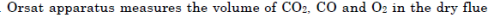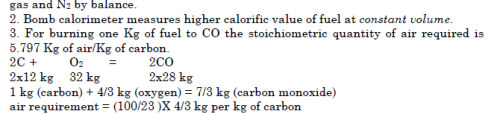QUESTION: 5

Which one of the following orders is the correct order of passing the flue gases through the different absorbents (in the flasks) during analysis in Orsat apparatus?

Solution:
QUESTION: 6

Orsat apparatus is used to determine products of

Solution:
QUESTION: 7

Items given in List I and List II pertain to gas analysis. Match List I with List II and select the correct answer.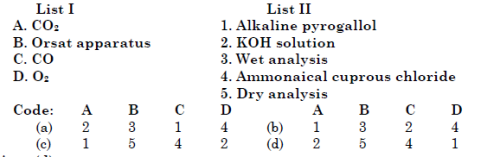Solution:
QUESTION: 8

In the Orsat flue gas analyser, ammoniacal cuprous chloride is used to absorb.

Solution:
QUESTION: 9

In Orsat apparatus

Solution:
QUESTION: 10

Consider the following statements:

1. For the combustion of pulverized coal, 5 to 10% excess air is required.

2. Air contains 21 % oxygen by weight.

3. The flue gases from a coal-fired furnace contain around 70% nitrogen byvolume.

4. In the combustion of liquid fuels the number of moles of the productsare invariably greater than the number of moles of the reactants.Of these statements

Solution:

Air contains 23 % oxygen by weight and 21 % oxygen by volume

QUESTION: 11

When solid fuels are burned, the nitrogen content of the flue gas byvolume is about

Solution:

when solid fuels are burnt, the nitrogen content of flue gas by volume is about 70%.

QUESTION: 12

Assertion (A): In constant pressure type gas turbines, large quantity of air is used,in excess of its combustion requirements.

Reason (R): Excess air is used to compensate for inevitable air-loss due to leakagesin the system.

Solution:
QUESTION: 13

The excess air required for combustion of pulverised coal is of the order of

Solution:

Greater surface area per unit mass of coal allows faster combustion reactions because more carbon becomes exposed to heat and oxygen, This reduces the excess air needed to complete combustion. The excess air required for combustion of pulverised coal is of the order of 5 to 10%

QUESTION: 14

Dry flue gases with a composition of CO2 = 10.4%, O2 = 9.6 and N2 = 80%,indicate that

Solution:

O2 = 9.6 means excess air is used

QUESTION: 15

Assertion (A): Excess air supplied to a combustor increases the efficiency ofcombustion

.Reason (R): Excess air tends to lower the temperature of the products of combustion.

Solution:

Excess air up to a limit increases efficiency, but beyond that the efficiency decreases for the reason given in R

QUESTION: 16

Consider the following statements:

1. Forward swept blade impeller is used in draft fans.

2. Forward swept blade impeller is used in room air-conditioners.

4. Forward swept blade impeller is used in exhaust fans.

Which of the statements given above is/are correct?

Solution:
QUESTION: 17

Forced draught fans of a large steam generator have

Solution:

Forced draught fans of a large steam generator have backward curved blades because these have steep head characteristics, good efficiency, high speed and ability to operate in parallel.

QUESTION: 18

At constant efficiency, the horse power of a fan is

Solution:
QUESTION: 19

Consider the following statements: Expansion joints in steam pipelines are installed to

1. Allow for future expansion of plant

2. Take stresses away from flanges and fittings

3. Permit expansion of pipes due to temperature rise.

Of these correct statements are

Solution:

Expansion joints in steel pipe lines are installed to take stresses away from flanges and fittings and also to permit expansion of pipes due to temperature rise.

QUESTION: 20

Which one of the following fuels is used to determine the water equivalent of a bomb calorimeter?

Solution:
QUESTION: 21

Amount of oxygen needed to completely burn l1lg of methane (CH4) is

Solution: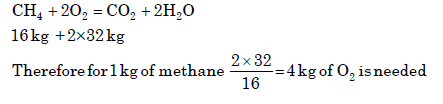QUESTION: 22

Which of the following combustion systems requires maximum excess air?

[IAS-2002]

Solution:

pulverized coal combustion required less amount of excess air as it burns fine particles.

QUESTION: 23

A double acting steam engine with a cylinder diameter of 19 cm and a stroke of 30 cm has a cut-off of 0.35. The expansion ratio for this engine is nearly

Solution: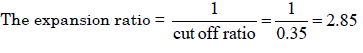QUESTION: 24

Match List I with List II and select the correct answer using the code given below the Lists: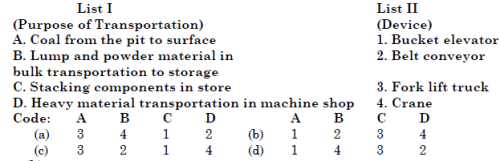Solution:
QUESTION: 25

Amount of oxygen needed to completely burn l1lg of methane (CH4) is

Solution: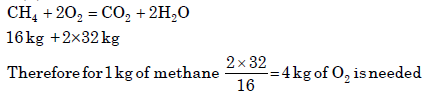QUESTION: 26

Assertion (A): The calorific value of a fuel depends on the excess air used for combustion.

Reason (R): The purpose of supplying excess air is to ensure complete combustion.

Solution:

A is false. Calorific value is the heat transferred when the product of complete combustion of a sample of fuel are cooled to the initial temperature of air and fuel. There is no relation with excess air.

QUESTION: 27

Inside a large power boiler, the flue gas pressure will be above the atmospheric pressure

Solution:
QUESTION: 28

An induced draft fan is normally located at the

Solution:
QUESTION: 29

A power plant consumes coal at the rate of 80 kg/s while the combustion air required is 11kg/kg of coal. What will be the power of the F.D. fan supplying the air, if its efficiency is 80% and it develops a pressure of 1.2 m of water? (Assume density of air to be 1.2 kg/m3)

Solution: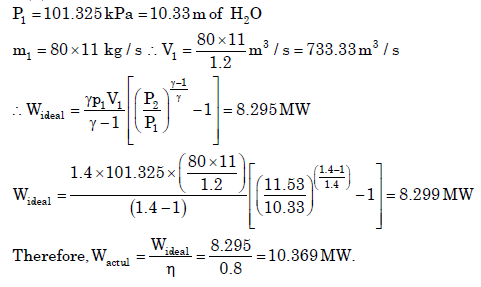QUESTION: 30

The p-V diagram for thereciprocating steam engineis shown in the figure. Thelength A-B represents the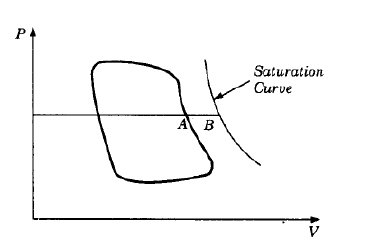Solution:

The length AB in the figure represents the missing quantity.